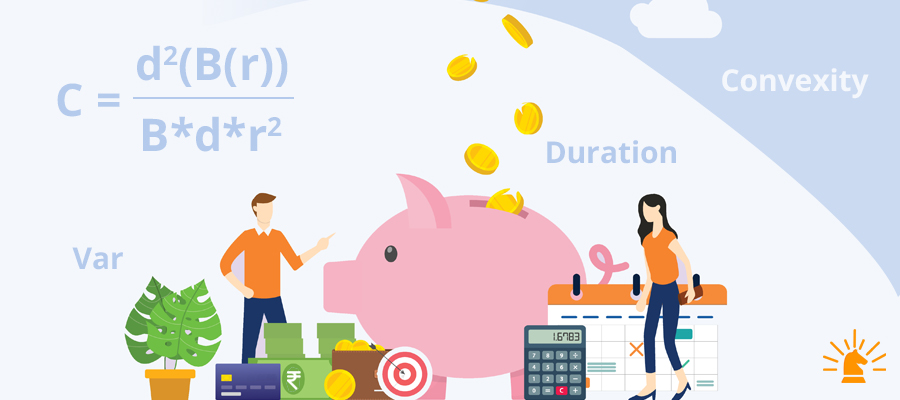## Fixed Income Mathematics

###### Updated on Oct 12, 2020 | By Author Sakshi ShekhawatTags:

The starting point for this analysis is the yield-to-maturity or internal rate of return on future cash flows. The return on a fixed-rate bond is affected by many factors, the most important of which is the receipt of the interest and principal payments in the full amount and on the scheduled dates.

Assuming no default, the return is also affected by changes in interest rates as that affects the reinvestment of the coupon amount received from the bond and the price of the bond if it is sold before it matures.

Measures of the price change can be derived from the mathematical relationship used to calculate the price of the bond.

The first of these measures is the duration. It estimates the change in the price for a given change in interest rates. The second measure is convexity. It takes into account the fact that the relationship between price and yield-to-maturity of a fixed-rate bond is not linear.

Let’s look at them one by one.

#### What is Duration?

The Duration of the bond refers to the price sensitivity of a bond to the corresponding change in interest rates. It is often mistaken with the term or time to the maturity of the bond.

It measures how many years it would take an investor to be repaid the bond’s price by its total cash flows.  In general, the higher the duration, the more a bond's price will drop as interest rates rise and the greater the interest rate risk.

As a general rule, interest rates are inversely proportional to the prices of the bond. So an increase in interest rate would lead to the proportional decline in the price of the bond for every year of duration.

Let’s understand it simply:

If a bond has a duration of 5 years and interest rates increase by 0.01%, the bond’s price will drop by 0.01%  multiplied by 5 years which is equal to 0.05%.

So, the Duration depends on two factors- time to maturity and the coupon rate.

Similarly, there is a term called PVBP. PVBP is the acronym for the Price Value of a Basis Point. It is used to measure or determine the change in the price of a given bond when there is a change of one basis point in its yield. It is a method to test the price sensitivity of a bond.

Drawn on a curve, it would be a downward sloping curve as price and yields are inversely proportional to each other. PVBP is nothing but the measure of the slope of this curve.

#### Convexity

Convexity refers to the interaction between the price of a bond and its yield, due to a corresponding change in the interest rates. The Duration has limitations because the given relationship is not linear in nature. In reality, it is convex.

Hence, convexity is used as a measure to gauge the curvature of the changes in price and yield relationship, in relation to the changes in interest rates.

The formula for Convexity is:

Where C is convexity, B is the bond price, r is the interest rate and d are duration

#### Value at Risk

Another common risk management tool is the Value at Risk (VaR).

Value at Risk (VaR) a statistical tool to measure and quantify financial risk within a firm or portfolio over a specific time frame. This metric is often used by Banks to determine the extent and probability of occurrence of a potential loss on the advances or investments. It is also widely used by risk managers to measure and control the level of risk exposure. It can be applied to a specific position or the whole portfolio. It is quite commonly used as a tool for Risk Management.

Imagine going to a store and asking the shopkeeper the price of water in terms of kilograms. Well, won’t be a pleasant answer for sure. Similarly, many investors, especially while starting out, do not try to understand the metrics for gauging fixed income securities. Instead, they use the same concepts from the equity markets, and more often than not, it does not end well for them. These are very different asset classes. Hence, it is extremely important to know these metrics in order to make educated decisions while making such investments.

Key Categories
Related Articles